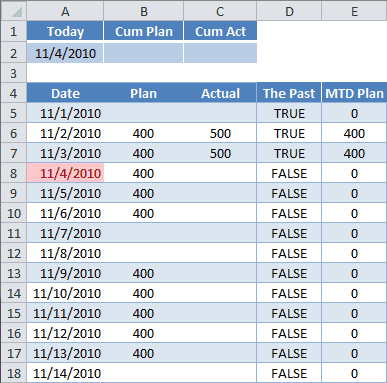# Multi-Cell Array Formula in Excel

Arrays are difficult to understand because Excel holds them internally and it’s hard to conceptualize how operations are performed on the data. I had a vexing problem that was solved by using a single-cell array formula and wanted to share it with you.

However, because of the subject nature of Arrays, I’m going to show the solution to this problem in two parts. Here I’ll cover a Multi-Cell Array formula and my next post will talk about a Single-Cell Array formula, which, turns out, is my magic formula.### The Problem

Here’s an example worksheet. The data here is rather simple: Date, Plan, and Actual. The Plan data is set, and Actual data is plugged in when completed.

My ultimate goal is to have a formula in cell B2 for the Cum Plan, which summarizes Plan history, i.e., for all dates in the past. And keep in mind I need the formula to work with earlier versions of Excel, so no SUMIFS availability.

I’ve added two columns that will hold multi-cell array formulas, The Past and MTD Plan. But first, for reasons that will become apparent later, I’ll cover plain, regular formulas for these two columns.

#### Some Groundwork

The formula in cell D5 uses a comparison operator (>). The formula is:

=(\$A\$2>A5)

The result is TRUE. \$A\$2 is an absolute reference to cell A2, where the TODAY() Function resides and merely shows today’s computer clock date. I could have entered =TODAY()>A5 in cell D5 with the same result. When the date for Today is less than or equal to the Date in column A, we get FALSE.TRUE and FALSE are binary values, where Excel equates TRUE = 1 and FALSE = 0. You can see this clearly by multiplying the previous formula by 1. (see picture)

=(\$A\$2>A5)*1

Any number multiplied by TRUE is like multiplying by 1, and any number multiplied by FALSE is like multiplying by zero (0).

The formula in column E for MTD Plan is to multiply The Past times Plan. The formula in cell E5 is:

=D5*B5

## Entering a Multi-Cell Array Formula

To enter any Array formula requires a special hand-shake, er, key press. You select multiple cells, type the formula, then hold the Ctrl+Shift keys down and press Enter.

The array formula in cells D5:D18 is entered by:

• Selecting cells D5:D18
• Typing the formula =\$A\$2>A5:A18
• Use keyboard combination Ctrl+Shift+Enter

Which gives you the following formula for the entire range:

{=\$A\$2>A5:A18}

The curly braces are the result of using the special keyboard combination: Ctrl+Shift+Enter. You don’t enter the curly braces, Excel does this automatically.

The array formula in cells E5:E18 is entered by:

• Selecting cells E5:E18
• Typing the formula =D5:D18*B5:B18
• Use keyboard combination Ctrl+Shift+Enter

Which gives you,

{=D5:D18*B5:B18}

in every cell of the range.

### Things to Know About a Muli-Cell Array Formula

• You must select all cells in the range before you enter the formula
• You can’t change one cell in a multi-cell array formula
• You can’t insert a row into the range of a multi-cell array formula
• To edit: select the range then press F2 (re-enter the array by Ctrl+Shift+Enter)
• To convert to a regular formula: select the range, press F2, then Ctrl+Enter
• To select the entire range: select a cell in the range, then press Ctrl+/

## Uses for a Multi-Cell Array Formula

I haven’t found the need to use a multi-cell array formula, except for a gateway to understanding a single-cell array. At least with a single-cell array you can use the Evaluate Formula dialog box to see how the Array is working in Excel’s internal memory.

Using some of the concepts I’ve introduced here, my next post will cover a Single-Cell Array Formula that solves my problem of getting one formula to show the Cumulative Plan quantity for all days before today. I promise it will be more satisfying than this post.## 4 thoughts on “Multi-Cell Array Formula in Excel”

1.Reuvain

In the sentence:

“And keep in mind I need the formula to work with earlier versions of Excel, so no SUMIF availability.”

I believe you meant SUMIFS, not SUMIF.

1.Gregory

You’re absolutely correct. My bad. I’ll have to update this post. Thanks for the comment.